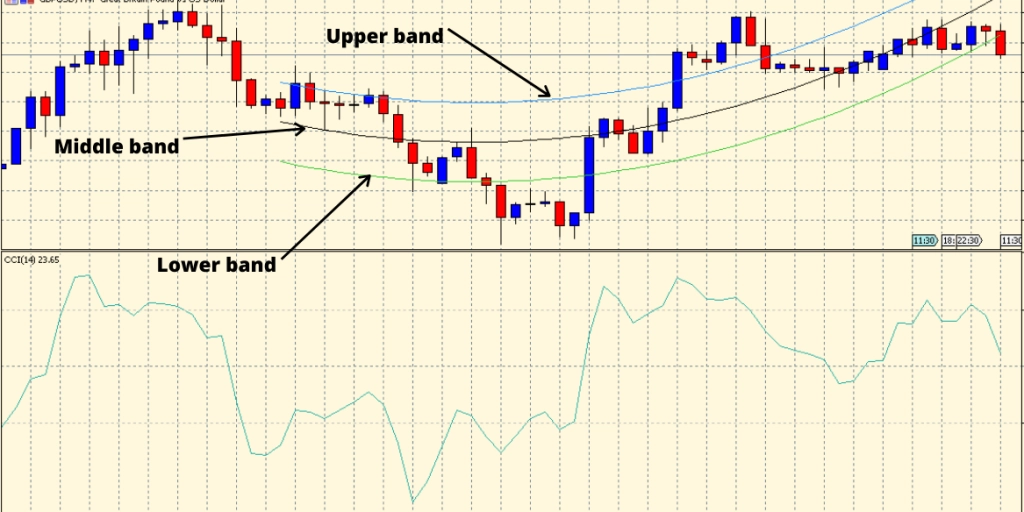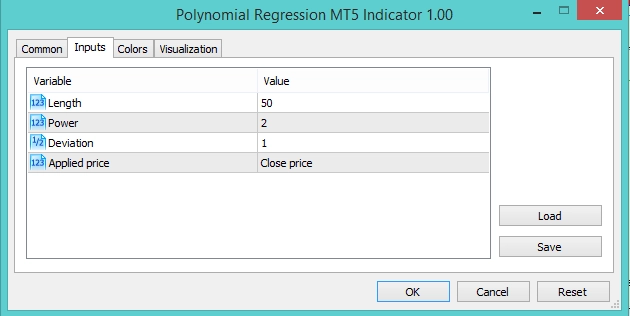## Polynomial Regression Indicator## \$20

The PRC (Polynomial Regression Channel) is a regression indicator that draws a line to fit best on the chart. It applies a polynomial function to linear regression function (three-line technical indicator used for analyzing upper and lower band limits of the trend) through recent period’s data. That’s why it is also called the Linear Regression Channel. As the Polynomial Regression Channel is a regression band, it adjusts itself for volatility.

## How to use the Polynomial Regression Channel?

As we mentioned, the Polynomial Regression Channel uses a degree of polynomial to display data points. This creates boundaries around the price. Here the support and resistance come into play from the bears and the bulls.

The bullish trend appears when the price is increasing, and the slope of the regression line is positive. This is known as a bullish regression channel.

The bearish trend appears when there is a decrease in price, and the slope of the regression line is negative. This is known as the bearish regression channel.

With the Polynomial Regression Channel, you have the upper band, the lower band, and the neutral band. The neutral band is present in the middle.To trade on the Polynomial Regression Channel, we need to keep an eye when the price interacts with one of these bands.

Each time the price hits the upper band or the lower band, this can be a signal to buy or sell. The bands adjust themselves depending on the uptrend and the downtrend.

You can choose your own settings for the indicator or author’s settings.## Polynomial Regression Channel Strategy

The basic strategy for Polynomial Regression Channel is to buy when the price is in the lower band and sell when the price is in the upper band. The trader may choose to exit when the price is in the middle band.

By using Polynomial Regression Channel in a trading strategy, traders can try to detect the trend’s direction. For example, when the price hits the upper band, this sometimes indicates the market is in a downtrend.

### Polynomial Regression Channel buy strategy

• The price should enter the lower band region.
• Wait for the price to close bullish before entering.
• Place the stop-loss near the swing low area.
• Exit the trade when CCI is above 50.